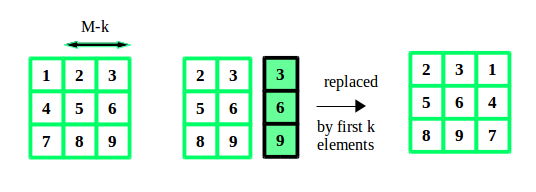GeeksforGeeks App
Open AppBrowser
Continue

# Python3 Program to Rotate the matrix right by K times

Given a matrix of size N*M, and a number K. We have to rotate the matrix K times to the right side.
Examples:

```Input :  N = 3, M = 3, K = 2
12 23 34
45 56 67
78 89 91

Output : 23 34 12
56 67 45
89 91 78

Input :  N = 2, M = 2, K = 2
1 2
3 4

Output : 1 2
3 4```

A simple yet effective approach is to consider each row of the matrix as an array and perform an array rotation. This can be done by copying the elements from K to end of array to starting of array using temporary array. And then the remaining elements from start to K-1 to end of the array.
Lets take an example:## Python3

 `# Python program to rotate``# a matrix right by k times` `# size of matrix``M ``=` `3``N ``=` `3``matrix ``=` `[[``12``, ``23``, ``34``],``          ``[``45``, ``56``, ``67``],``          ``[``78``, ``89``, ``91``]]` `# function to rotate``# matrix by k times``def` `rotateMatrix(k) :` `    ``global` `M, N, matrix``    ` `    ``# temporary array``    ``# of size M``    ``temp ``=` `[``0``] ``*` `M``    ` `    ``# within the size``    ``# of matrix``    ``k ``=` `k ``%` `M``    ` `    ``for` `i ``in` `range``(``0``, N) :``    ` `        ``# copy first M-k elements``        ``# to temporary array``        ``for` `t ``in` `range``(``0``, M ``-` `k) :``            ``temp[t] ``=` `matrix[i][t]``    ` `        ``# copy the elements from``        ``# k to end to starting``        ``for` `j ``in` `range``(M ``-` `k, M) :``            ``matrix[i][j ``-` `M ``+` `k] ``=` `matrix[i][j]``    ` `        ``# copy elements from``        ``# temporary array to end``        ``for` `j ``in` `range``(k, M) :``            ``matrix[i][j] ``=` `temp[j ``-` `k]``    ` `# function to display``# the matrix``def` `displayMatrix() :` `    ``global` `M, N, matrix``    ``for` `i ``in` `range``(``0``, N) :``    ` `        ``for` `j ``in` `range``(``0``, M) :``            ``print` `(``"{} "` `.``                   ``format``(matrix[i][j]), end ``=` `"")``        ``print` `()` `# Driver code``k ``=` `2` `# rotate matrix by k``rotateMatrix(k)` `# display rotated matrix``displayMatrix()` `# This code is contributed by``# Manish Shaw(manishshaw1)`

Output:

```23 34 12
56 67 45
89 91 78```

Time Complexity: O(N*M)
Auxiliary Space: O(M)

Please refer complete article on Rotate the matrix right by K times for more details!

My Personal Notes arrow_drop_up